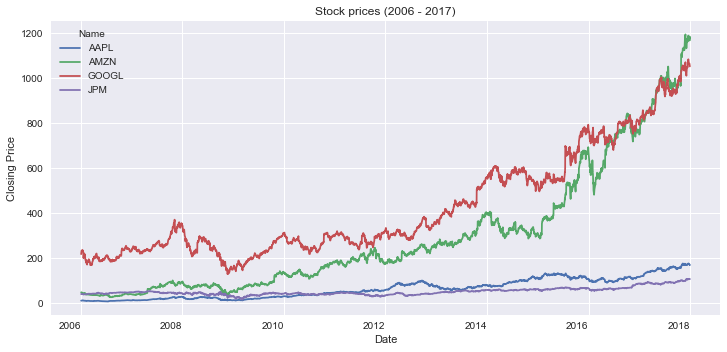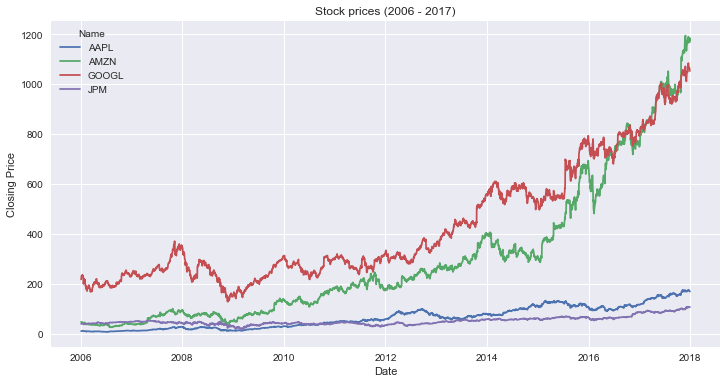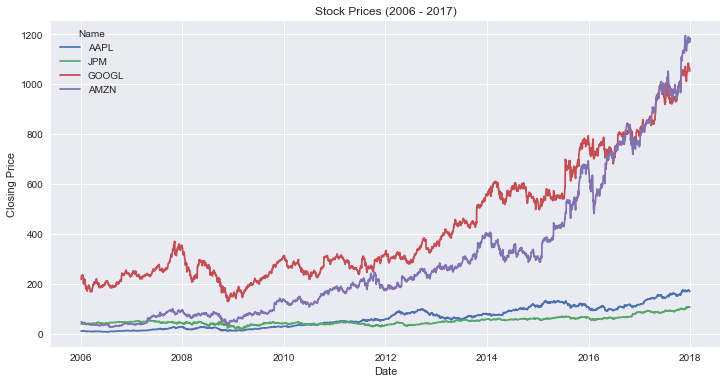# Python Data Viz Libraries Compared: 8 Popular Graphs Made with pandas, matplotlib, seaborn, and plotly.express¶

Author: Dylan Castillo

I'm teaching a course about the essential tools of Data Science. Among those, I'm going to cover how to use some of the most popular data visualization libraries in Python: pandas (yes, that's not a typo!), matplotlib, seaborn, and plotly.express.

I thought it be useful for my students to have cheat sheet with some popular graphs made with each of these tools. So I wrote this cheat sheet.

In the next sections, you'll learn how to set up your local environment, read the data, and get the code to make the following types of graphs:

• Line plot
• Grouped bars plot
• Stacked bars plot
• Area chart
• Pie/Donut chart
• Histogram
• Scatter plot
• Boxplot

Let me know what you think!

## Set Up a Virtual Environment¶

Working with virtual environments will save you lots of headhaches when working in Python project. So, you'll start by creating one, and installing the required libraries.

If you're using venv, then here's how you set up your local enviroment:

$python3 -m venv .dataviz$ source .dataviz/bin/activate
(.dataviz) $python3 -m pip install pandas==1.2.4 numpy==1.2.0 matplotlib==3.4.2 plotly==4.14.3 seaborn==0.11.1 notebook==6.4.0 (.dataviz)$ jupyter notebook

If you're using conda, then you need to run these commands:

$conda create --name .dataviz$ conda activate .dataviz
(.dataviz) $conda install pandas==1.2.4 numpy==1.19.2 matplotlib==3.4.2 plotly==4.14.3 seaborn==0.11.1 notebook==6.4.0 -y$ jupyter notebook

That's it! These commands will:

1. Create a virtual environment called .dataviz
2. Active the virtual environment
3. Install the required packages (pandas, numpy, matplotlib, plotly, seaborn, and notebook)
4. Start a Jupyter Notebook

Note that if you're only planning on using just one of the data visualization libraries, then feel free not to install all of them. For example, if you want to use plotly.express, you don't need to install matplotlib and seaborn.

## Start Jupyter Notebook and Import Libraries¶

Open Jupyter Notebook. Then, create a new notebook by clicking on New > Python3 notebook in the menu. By now, you should have an empty Jupyter notebook in front of you. Now, let's get to the fun part!

First, you'll need to import the required libraries. Create a new cell in your notebook and paste the following code to import the required libraries:

In :
# All
import pandas as pd
import numpy as np

# matplotlib
import matplotlib.ticker as mtick
import matplotlib.pyplot as plt

# plotly
import plotly.io as pio
import plotly.express as px

# seaborn
import seaborn as sns

# Set templates
pio.templates.default = "seaborn"
plt.style.use("seaborn")


This code will import the required libraries and set up the themes for matplotlib and plotly. Each library provides you with a specific set of functionalities:

• pandas helps you read the data
• matplotlib.pyplot, plotly.express and seaborn will help you make the graphs
• matplotlib.ticker provides with a way to set specific settings of the tickers on your axes in your matplotlib graphs
• plotly.io makes it easy to define a specific theme for your plotly graphs

In lines 17 and 18, you define the themes for plotly and matplotlib. In this case, you set them to use the seaborn theme. This will make the graphs from all the libraries look similar.

## Understand the Data¶

Throughout this tutorial you'll use a dataset with stock market data for 29 companies compiled by ichardddddd. It has the following columns:

• Date: Date corresponding to observed value
• Open: Price (in USD) at market open at the specified date
• High: Highest price (in USD) reached during the corresponding date
• Low: Lowest price (in USD) reached during the corresponding date
• Close: Price (in USD) at market close at the specified date
• Volume: Number of shares traded
• Name: Stock symbol of the company

You can take a look ad the data by taking a sample of a few rows:

In :
url = "https://raw.githubusercontent.com/szrlee/Stock-Time-Series-Analysis/master/data/all_stocks_2006-01-01_to_2018-01-01.csv"
df.sample(5)

Out:
Date Open High Low Close Volume Name
25931 2013-01-18 52.24 52.34 51.81 52.34 8492176 DIS
53204 2013-06-05 98.13 98.16 96.12 96.42 5394802 MCD
39946 2008-09-26 117.21 121.01 117.01 119.42 4760683 IBM
37191 2009-10-15 27.28 27.37 27.05 27.30 13350145 HD
2877 2017-06-08 204.84 206.03 204.09 205.94 2451348 MMM

This is a long dataset (in regards to the stock names). In the next sections, you'll notice that some libraries make it easy to work with data in this form, and others will require you to transform it into a wide dataset.

That's it! Now you can find whatever graph you'd like to make and copy-paste its code.

## Line Plot¶

In :
url = "https://raw.githubusercontent.com/szrlee/Stock-Time-Series-Analysis/master/data/all_stocks_2006-01-01_to_2018-01-01.csv"

df = df.loc[df.Name.isin(["AAPL", "JPM", "GOOGL", "AMZN"]), ["Date", "Name", "Close"]]
df["Date"] = pd.to_datetime(df.Date)
df.rename(columns={"Close": "Closing Price"}, inplace=True)


### Line Plot Using pandas¶

In :
df_wide = df.pivot(index="Date", columns="Name", values="Closing Price")
df_wide.plot(
title="Stock prices (2006 - 2017)", ylabel="Closing Price", figsize=(12, 6), rot=0
)

Out:
<matplotlib.axes._subplots.AxesSubplot at 0x16d5f6ac0>### Line Plot Using matplotlib¶

In :
fig, ax = plt.subplots(figsize=(12, 6))

for i, g in df.groupby("Name"):
ax.plot(g["Date"], g["Closing Price"], label=i)

ax.set_title("Stock prices (2006 - 2017)")
ax.set_ylabel("Closing Price")
ax.set_xlabel("Date")
ax.legend(title="Name")

Out:
<matplotlib.legend.Legend at 0x16d714400>### Line Plot Using seaborn¶

In :
fig, ax = plt.subplots(figsize=(12, 6))
sns.lineplot(data=df, x="Date", y="Closing Price", hue="Name", ax=ax)
ax.set_title("Stock Prices (2006 - 2017)")

Out:
Text(0.5, 1.0, 'Stock Prices (2006 - 2017)')### Line Plot Using plotly.express¶

In :
fig = px.line(
df,
x="Date",
y="Closing Price",
color="Name",
title="Stock Prices (2006 - 2017)",
width=900,
height=500,
)
fig.show()